Type Here to Get Search Results !

# PHYSICS FORM TWO TOPIC 4: FORCES IN EQUILIBRIUMFORCES IN EQUILIBRIUM
The Effects of Turning Forces
Explain the effects of turning forces
force is a push or pull which when applied to a body it will change its state either by stopping it if it was in motion or making it move if it was at rest.
If a body under the action of a net external force is allowed to rotate about a pivot, the body will tend to turn in the direction of the applied force.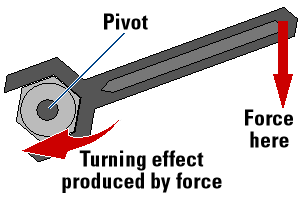Examples of turning effect of force:
• A person pushing a swing will make the swing rotate about its pivot.
• A worker applies force to a spanner to rotate a nut.
• A person removes a bottle’s cork by pushing down the bottle opener’s lever.
• Force is applied to a door knob and the door swings open about its hinge.
• A driver can turn a steering wheel by applying force on its rim.
The Moment of Force
Determine the moment of force
Moment of forceabout a point is the turning effect of the force about that point. The change of state of a body can appear in several forms and the most common form is the turning effect which is referred to as moment of a force.
The unit for a force isNewton, Nwhile that for moment of a force isNewton-meter, Nm.
Moment = Force, F x Perpendicular distance, x.
Moment = Fx
Consider the diagram below;Moment of W₁ about the fulcrum. = W₁d₁
Moment of W₂ about fulcrum = W₂d₂
The Principle of Moments
State the principle of moments
The Principle of moments states that:”If a body is in equilibrium under a force which lie in one plane, the sum of the clockwise moments is equal to the sun of the anticlockwise moments about any point in that plane.”
Activity 1
Experiment.
Aim: To determine the moment of a force.
Materials and apparatus:Meter rule, several different weights, inelastic cotton thread, knife edge and a marker pen.
Procedures
• Balance the meter rule horizontally on a knife edge.
• Mark a balance point as C. Use the marker pen to do that.
• Suspend a meter rule from a fixed axis through C. Suspend unequal weights W₁ and W₂ from the meter rule by using thin cotton threads.
• Adjust the distance d₁ and d₂ of the weights W₁ and W₂ from C until the meter rule balance.
• Repeat the experiment five times using different values of W₁ and W₂.Record the results on the table as shown below.
 W₁(g) W₂(g) d₁(cm) d₂(cm) W₁ d₁ (gcm) W₂ d₂ (gcm)
Observation:In each case it will be found that W₁ d₁ is equal to W₂ d₂.
The Principle of Moment in Daily Life
Apply the principle of moment in daily life
Moment of a force is used in the following:
• Is applied by a hand to unscrew a stopper on the bottle.
• Is applied by a spanner to unscrew a nut on a bottle.
• Turning a steering wheel of a car.
Centre of Gravity
Explain centre of gravity
Center of gravity of a body is the point at which the weight of a body appears to be concentrated. OR center of gravity of a body is the point of application of the resultant force due to the earth attraction on the body.The center of gravity of a regular body is found to be at its geometrical center.
Example 1
1. Centre of gravity of a uniform meter rule is at the 50cm mark.
2. Centre of gravity of a circular object is at its center.
The center of gravity of irregular bodies can be found experimentally.
Centre of Gravity of Regular Shaped Body
Determine centre of gravity of regular shaped body
Activity 2
Experiment.
Aim; To determine the center of gravity of a regular body (meter rule)
Materials and apparatus: Meter rule, a known weight and a spring balance.
Procedures
• Balance the meter rule on a fulcrum and mark the position where the meter rule balances horizontally by the letter G.
• Hang a known weight from one end of the meter rule and determine the position where the meter rule balances and mark it X.
• Measure the distance XC and XG(C is the point at the end of the meter rule where an object of known weight, w is attached).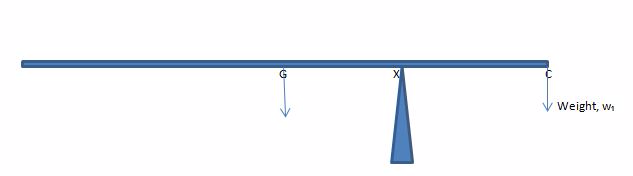The weight, w of the meter rule always acts at G downwards. Thus it will create a moment to balance the moment due to w.
Results
The mass of the meter rule, w is determined by using the principle of moments.Taking moments about X;
Clockwise moments = Anticlockwise moments
w₁ .XC =w. XG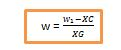Centre of Gravity of Irregular Shaped Body
Determine centre of gravity of irregular shaped body
Activity 3
Experiment
Aim: To determine the center of gravity of irregular plate.
Materials and apparatus:Card board, plumb line, inelastic cotton thread, clamp, clamp rod and a marker pen.
Procedures
• Make an irregular shaped piece of card board.
• Make three small holes well-spaced round the edge of the cardboard.
• Suspend the cardboard using a nail or the rod of a clamp or a stout pin in a clamp from one of the holes.
• Suspend the plumb line in front of the card.
• Mark the position of the string of the plumb line on the card by two small crosses.
• Repeat the exercise for the other two holes. Join each pair of crosses with a straight line.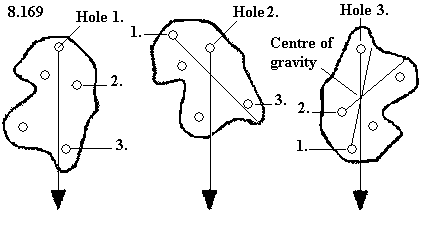Result and Observation
The three straight lines meets at a certain point. This point is the center of gravity of the body.
The Condition for Equilibrium
Explain the condition for equilibrium
Equilibrium:A body is said to be balanced if its center of gravity is directly above the point of support.
Stable, Unstable and Neutral Equilibrium
Explain Stable, Unstable and Neutral equilibrium
There are three types of equilibrium, namely:
1. Stable equilibrium
2. Unstable equilibrium
3. Neutral equilibrium
Stable equilibrium:A body is said to be in stable equilibrium if is given with small displacement the center of gravity will be raised and the body returns to its original position after displacement.
Unstable equilibrium:A body is said to be in unstable equilibrium if when given a small displacement the center of gravity will be lowered and the body doesn’t returns to its original position after displacement.
Neutral equilibrium:A body is said to be in neutral equilibrium when a small displacement doesn’t alter the position of the center of gravity; the body is at rest in whichever position it is placed, eg, rolling a sphere or a barrel.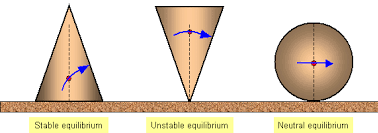Conditions of Stable, Unstable and Neutral Equilibrium in Daily Life
Apply conditions of stable, unstable and neutral equilibrium in daily life
Application of turning effect in daily life
• Is applied by a hand to unscrew a stopper on the bottle.
• Is applied by a spanner to unscrew a nut on a bottle.
• Turning a steering wheel of a car.
Exercise 1
.The moment of a force about a point is 1120 Nm.If the magnitude of the force is 5600N,find the perpendicular distance between the point and the line of action of the force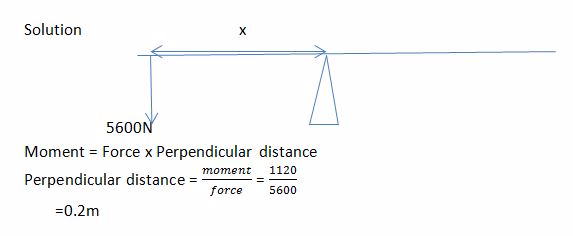Tags